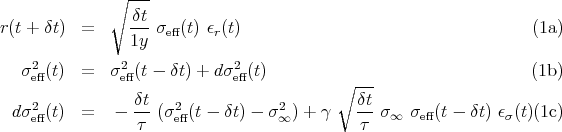Base information

### Heston process with normal innovations

The Heston model is a stochastic volatility process where the noise term for the variance dynamic is modulated by the volatility. The equations are:where σeff2(t) is an annualized variance, σ 2 fixes the mean annualized variance, τ sets the mean reversion time to the mean variance, and γ is the mean noise level. The main interest of the model is that an exact solution for the European option price can be computed when the residues ϵr and ϵσ are Gaussian, see Heston and Nandi .

The parameters for the simulations are:

• σ = 0.11.
• τ = 4 days.
• γ = 1.65
• p(ϵr), p(ϵσ): normal distributions.

The simulation time corresponds to 200 years with a time increment δt = 3 minutes.

### References

S. Heston and S. Nandi. A closed-form GARCH option pricing model. Review of Financial Studies, 13:585–626, 2000.